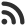Feed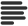Articles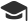Tutorials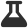CodingLab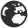CommunityLeaderboardharsh_shukla published an article 2 months ago

# Minimum steps to reach end of array under constraints

Given an array of one digit numbers. Your task is to find out the minimum number of steps that is needed to reach the end of the array when you are at the first index of the array in the begining. In one steps, you can jump to the neighbor indices or you can jump to the position whose value is same as the value of your curret index.

That means, if you are at i, then in one step you can reach to, arr[i-1] or arr[i+1] or arr[K] if arr[K] = arr[i] ( value of arr[K] is same as arr[i] )

Let's take a look at examples:

Input

``arr[] = {5, 4, 2, 5, 0}``

Output

``2``

Explanation

We start from 5(0), in first step jump to next 5 and in second step we move to value 0 (End of arr[]). Here we took 2 steps so 2 will be the output.

Input

``arr[] = [0, 1, 2, 3, 4, 5, 6, 7, 5, 4, 3, 6, 0, 1, 2, 3, 4, 5, 7]``

Output

``5``

Explanation

0(0) -> 0(12) -> 6(11) -> 6(6) -> 7(7) -> (18)

We take total 5 steps, so 5 will be the output.

Apporach

This problem can be solved using BFS. We can consider the given array as unweighted graph where every vertex has two edges to next and previous array elements and more edges to array elements with same values. Now for fast processing of third type of edges, we keep 10 vectors which store all indices where digits 0 to 9 are present. In above example, vector corresponding to 0 will store [0, 12], 2 indices where 0 has occurred in given array.

Another Boolean array is used, so that we don’t visit same index more than once. As we are using BFS and BFS proceeds level by level, optimal minimum steps are guaranteed.

Implementation

``````// C++ program to find minimum steps to reach end of array
#include <bits/stdc++.h>
using namespace std;

// returns minimum step to reach end of array

int getMinStepToReachEnd(int arr[], int N)
{
// visit boolean array checks whether current index
// is previously visited
bool visit[N];

// distance array stores distance of current
// index from starting index
int distance[N];

// digit vector stores indicies where a
// particular number resides
vector<int> digit;

// In starting all index are unvisited
memset(visit, false, sizeof(visit));

// storing indices of each number in digit vector
for (int i = 1; i < N; i++)
digit[arr[i]].push_back(i);

// for starting index distance will be zero
distance = 0;
visit = true;

// Creating a queue and inserting index 0.
queue<int> q;
q.push(0);

// loop untill queue in not empty
while(!q.empty())
{
// Get an item from queue, q.
int idx = q.front();	 q.pop();

// If we reached to last index break from loop
if (idx == N-1)
break;

// Find value of dequeued index
int d = arr[idx];

// looping for all indices with value as d.
for (int i = 0; i<digit[d].size(); i++)
{
int nextidx = digit[d][i];
if (!visit[nextidx])
{
visit[nextidx] = true;
q.push(nextidx);

// update the distance of this nextidx
distance[nextidx] = distance[idx] + 1;
}
}

// clear all indices for digit d, because all
// of them are processed
digit[d].clear();

// checking condition for previous index
if (idx-1 >= 0 && !visit[idx - 1])
{
visit[idx - 1] = true;
q.push(idx - 1);
distance[idx - 1] = distance[idx] + 1;
}

// checking condition for next index
if (idx + 1 < N && !visit[idx + 1])
{
visit[idx + 1] = true;
q.push(idx + 1);
distance[idx + 1] = distance[idx] + 1;
}
}

// N-1th position has the final result
return distance[N - 1];
}

// Main function
int main()
{
int arr[] = {0, 1, 2, 3, 4, 5, 6, 7, 5, 4, 3, 6, 0, 1, 2, 3, 4, 5, 7};
int N = sizeof(arr) / sizeof(int);
cout << getMinStepToReachEnd(arr, N);
return 0;
}
``````

``5``

 arrow_upward UpvoteComment arrow_downward downvote

Events

Nov. 28, 2018, 5:30 p.m.

Python from zero to hero

place Delhi

Aug. 13, 2018, 5:30 p.m.

Python from zero to hero

place Bangalore ( HackersFriend office BTM Layout)